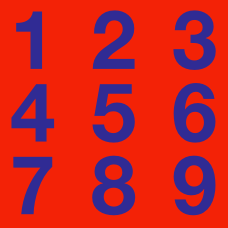Number Theory

# Number Theory Warmups: Level 4 Challenges

What is the remainder when $1^{2013}+2^{2013}+\cdots +2012^{2013}+2013^{2013}$ is divided by $2014$?

$\large \frac{1}{a}+\frac{1}{b}=\frac{1}{100000}$

How many distinct ordered pairs of positive integers $(a,b)$ are there which satisfy the above equation?

$\large \displaystyle \dfrac{a}{\frac{b}{c}} = \dfrac{\frac{a}{b}}{c}$

The above equation is a common mistake made when interpreting fractions.

How many ordered triplets of integers ${(a,b,c)}$ with $-10\leq a,b,c \leq 10$ are there, such that the above equation is a true statement?

Given a positive integer $n$, let $p(n)$ be the product of the non-zero digits of $n$. (If $n$ has one digit, then $p(n)$ is equal to that digit.) Let

$S = p(1) + p(2) + \cdots + p(999).$

What is the largest prime factor of $S$?

$a, b$ and $c$ are distinct positive integers strictly greater than 1. If $abc$ divides $(ab-1)(bc-1)(ca-1)$, what is the value of $abc$?

×